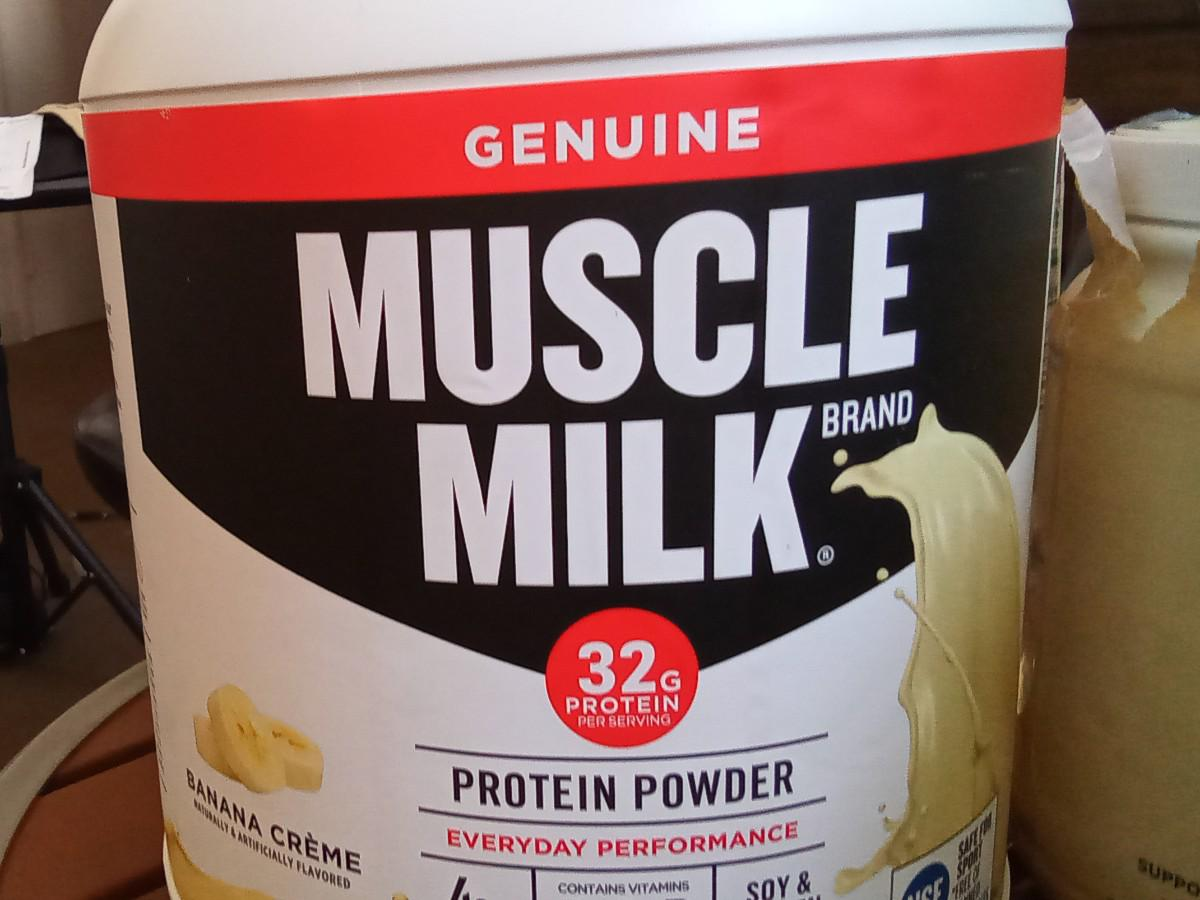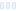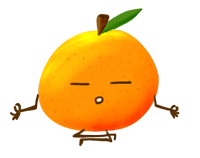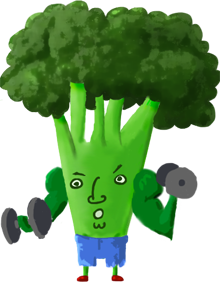# Lean Muscle Formula

## Banana Creme - Muscle Milk

Main info:

Lean Muscle Formula
Banana Creme - Muscle Milk
2 scoops
300.0 Calories
16.0 g
12.0 g
32.0 g

5.0 g
15.0 mg
6.0 g
240.0 mg
0 g
0 g

Percent calories from...
Nutrition Facts
For a Serving Size of (g)
How many calories are in Lean Muscle Formula? Amount of calories in Lean Muscle Formula: Calories Calories from Fat (%)
% Daily Value *
How much fat is in Lean Muscle Formula? Amount of fat in Lean Muscle Formula: Total Fat
How much saturated fat is in Lean Muscle Formula? Amount of saturated fat in Lean Muscle Formula: Saturated fat
How much cholesterol is in Lean Muscle Formula? Amount of cholesterol in Lean Muscle Formula: Cholesterol
How much sodium is in Lean Muscle Formula? Amount of sodium in Lean Muscle Formula: Sodium
How much potassium is in Lean Muscle Formula? Amount of potassium in Lean Muscle Formula: Potassium
How many carbs are in Lean Muscle Formula? Amount of carbs in Lean Muscle Formula: Carbohydrates
How many net carbs are in Lean Muscle Formula? Amount of net carbs in Lean Muscle Formula: Net carbs
How much fiber is in Lean Muscle Formula? Amount of fiber in Lean Muscle Formula: Fiber
How much glucose is in Lean Muscle Formula? Amount of glucose in Lean Muscle Formula: Glucose
How much protein is in Lean Muscle Formula? Amount of protein in Lean Muscle Formula: Protein
Vitamins and minerals
How much Vitamin A is in Lean Muscle Formula? Amount of Vitamin A in Lean Muscle Formula: Vitamin A
How much Vitamin C is in Lean Muscle Formula? Amount of Vitamin C in Lean Muscle Formula: Vitamin C
How much Calcium is in Lean Muscle Formula? Amount of Calcium in Lean Muscle Formula: Calcium
How much Iron is in Lean Muscle Formula? Amount of Iron in Lean Muscle Formula: Iron
Fatty acids
Amino acids
* The Percent Daily Values are based on a 2,000 calorie diet, so your values may change depending on your calorie needs.Loading similar foods...
Note: Any items purchased after clicking our Amazon buttons will give us a little referral bonus. If you do click them, thank you!Be cool

- Zen orangeI never skip arm day

- Buff broccoli